# The Definitive Guide to Microsoft Excel for Real Estate (Updated Dec 2021)

Microsoft Excel is the primary tool used by real estate financial modeling professionals. Even while numerous non-Excel alternatives have attempted to de-throne Excel, the 35+ year-old software has shown to be surprisingly resilient to competition. And thus, if you intend to work in real estate you must be proficient in Excel.

So what does it mean to be proficient in Excel as it relates to real estate financial modeling? Proficiency in this context means knowing how to use the Excel functions and features common to real estate financial modeling. In the A.CRE Definitive Guide to Microsoft Excel for Real Estate, I will teach you those Excel functions and features.

Are you an Accelerator member? A recommended pre-requisite to the Accelerator is having a basic understanding of Microsoft Excel. Various Accelerator members have asked for help becoming proficient in Excel as they begin the program. This resource is in response to that request. Not yet an Accelerator member? Consider joining today.### Before You Get Started – Your Excel Knowledge Today

While this guide teaches you the Excel functions and features you must master to be successful in real estate financial modeling, it nevertheless assumes you have at least a basic working knowledge of Microsoft Excel. If you’ve never opened an Excel workbook or you’re unfamiliar with the concept of cell formatting, that’s okay! I’ll point you to a resource that will get you up to speed.

But at this point, you should know at least the following Microsoft Excel basics before proceeding with this guide:

• What version of Excel you’re using (Important: make sure you’re using Excel 2013 or newer when modeling real estate)
• How to open, create, and save Workbooks
• The different between a Worksheet and a Workbook
• What a cell is, and how to insert, delete, drag, drop, cut, copy, paste and otherwise manipulate the contents of cells
• The difference between a column and row, and how to insert, delete, and otherwise modify columns and rows
• The various number, date, and text formats and how to change cell formats
• How to edit page layout, create print ranges, and print worksheets
• A cursory understanding of cell references and creating formulas using cell references
• How Excel functions work

If any or all of the above is new to you, I recommend you complete an Excel basics course. There are dozens of free options available online. My favorite is produced by a non-profit affiliate of the Goodwill Community Foundation called GCF Learn Free. Their Excel 2016 tutorial series is concise and professionally produced. I highly recommend it.

• Click here to take the free GCF Learn Free Excel 2016 basics course

## Introduction to Microsoft Excel for Real Estate

I should first point out that being an Excel expert and being a real estate financial modeling expert are not one and the same. It is true that most real estate financial modeling experts are also Excel experts. But being an expert at Excel does not mean you know how to model real estate cash flows. It simply means you know how to use a spreadsheet tool that just so happens to be the most common tool used by real estate financial modeling experts.

It’s also important to note that not all real estate financial modeling experts are Excel experts. Many professionals prefer to model real estate cash flows in other spreadsheet solutions such as Google Sheets, or in non-spreadsheet tools such as applications written in Python.

Thus, learning to model real estate cash flows (i.e. what is taught in our Accelerator program) is different entirely than learning to use Microsoft Excel. Excel just happens to be the most common medium for modeling real estate cash flows, but it’s not the only medium nor even the best medium.

Many of you know that I’m the Head of Investments and member of the founding team at Stablewood Properties. We’re an institutionally-backed, data-infused real estate operator that currently acquires STNL real estate assets around the United States. We rely on large swaths of data, together with speed and efficiency to quickly and accurately identify and underwrite assets as they come to market. To make that possible, we’ve built our own proprietary, non-Excel based underwriting application.

What’s interesting about our process of building the underwriting application, is that we first started in Excel. Excel acted as the communication conduit between the real estate financial modeling experts on our team, and the programming experts and data scientists ultimately building the application. And so even in the most advanced real estate firms in the world, at least as it relates to data and technology, Microsoft Excel plays an important part.

## Part I – The Only Excel Functions You’ll Ever Need to Analyze Real Estate

That’s a lofty statement: the only Excel functions you’ll ever need to analyze real estate, but it’s true.

In preparing for this guide, I scoured the 70+ real estate financial models in our Library. These models handle nearly every scenario you may come across in commercial and residential real estate. From those models, I pulled out every Excel function used and created a list.

The resulting list is exhaustive. While there are duplicative functions that I’ve left out for efficiency’s sake (e.g. INDEX/MATCH instead of VLOOKUP), in my view the Excel functions taught in this guide are the only functions you’ll ever need to learn to model real estate.

Definition: “An Excel Function is a predefined formula that performs calculations using specific values in a particular order.” The terms Function and formula are used interchangeable here, as well as in the industry.

In the following subsections, I’ll teach you how to use each of the Excel Functions in that list. If you master these functions, together with the basics in the introduction above and the Excel Features section below, you are ready to model real estate in Excel.

### i. ADDITION, SUBTRACTION, MULTIPLICATION, AND DIVISION LOGIC

I hesitate to even include these basic mathematical equations, as they’re so obvious (and basic). But no definitive guide is complete without them, and they are the most commonly used formulas you will use in Excel. So get used to using these in Excel.

I don’t believe these concepts need any further discussion, so allow me to go straight to the video.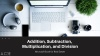### ii. SUM() AND AVERAGE() FUNCTIONS

The next functions are some of the most common functions used in Excel: SUM() and AVERAGE(). As their names describe, the SUM() function returns to sum of the values in a range of cells, while the AVERAGE() function returns the simple average of the values in a range of cells.

The syntax for SUM() and AVERAGE() functions is as follows:

SUM(number1,[number2],…)

AVERAGE(number1,[number2],…)

There are two options for writing these formulas. The first is to separate each value with a comma (e.g. number 1, number 2, number 3, etc). The second is to reference a range of cells, with each range separate by a comma.

So for instance, if you wrote =SUM(A1:A10), or you wrote =SUM( A1, A2, A3, A4, A5, A6, A7, A8, A9, A10), or you wrote =SUM(A1:A5, A6:A10), you would get the same result.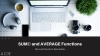### iii. TRUE AND FALSE LOGIC

With the basics needed to add, subtract, multiple, divide, and calculate average out of the way, let’s now turn to TRUE and FALSE logic in Excel.

TRUE and FALSE logic is the foundation of the conditional logic you’ll use in real estate financial modeling. Most every statement has either a TRUE or FALSE result, and that TRUE or FALSE result can be used to build some very basic but powerful formulas.

This form of logic is called Boolean logic, a concept I’ve explored at A.CRE before. When a logic statement has an outcome that is TRUE, Excel assigns a value of 1 to that statement. Whereas, when a logic statement has an outcome that is FALSE, Excel assigns a value of 0 to that statement. In computer science terms, Boolean Logic is:

“a form of algebra in which all values are reduced to either TRUE or FALSE. Boolean logic is especially important for computer science because it fits nicely with the binary numbering system, in which each bit has a value of either 1 or 0. Another way of looking at it is that each bit has a value of either TRUE or FALSE.”

I won’t go too far into the various ways in which Boolean Logic can be used in real estate financial modeling, other than to recommend that you begin thinking about the formulas you write in Excel as true and false statements.

Thus, if you write the formula =(2 = 2), Excel will spit out TRUE, whereas if you write the formula = (2=1), Excel will spit out FALSE.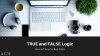### iv. IF() FUNCTION

With the concept of TRUE and FALSE discussed, let’s now look at the most commonly used logic statement in Excel.

Now the reason the IF() function is the most commonly used is not because it is the ideal solution in most cases. It is the most commonly used because it is the most intuitive logic function beginners. And since old habits die hard, as beginners advance in their modeling capabilities, the use of the IF() statement persists.

It’s important as you develop your modeling skills, that you use the simplest (i.e. easiest to follow) formulas/functions for the task. Sometimes that will be an IF() statements, but many times it won’t be. And so it’s important that you learn IF(), together with alternatives to IF().

In terms of the syntax for the IF() function:

IF(logical_test, value_if_true, [value_if_false])

To write an IF() statement, you start with a “logical test”. That logical test will either result in a TRUE or a FALSE outcome.

If the logical test results in TRUE, the IF() function will output the value (or reference) you enter in the second part of the formula. Otherwise, the IF() function will output the value (or reference) you enter in the third part of the formula.

NestedIF Statements

Now a section on IF() statements wouldn’t be complete without discussing nested IF statements. A nested IF statement is a formula in which you embed multiple IF() functions. So for instance, the syntax for a nested IF statement with two IF() functions is:

IF(logical_test, value_if_true, IF(logical_test, value_if_true, [value_if_false])

Truly complex (i.e. difficult to follow) IF() statements generally rely on nested IF statements. And this is where beginners truly get tripped up. They overly relying on one logic statement stacked on top of another logic statement in a nested IF formula to return a result, where a simpler function would do.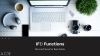### v. AND() & OR() FUNCTIONS

The next two logic statements are cousins of the IF() statement, in the sense that they can often accomplish the same thing as a nested IF without the complexity. The AND() function and the OR() function always yield either a TRUE or FALSE result. How you use the resulting TRUE or FALSE depends on the situation.

One common use of these functions is to include them in an IF() statement as part of the logical test. In the case of the AND() function, you might ask if two tests are both TRUE, if so then return X, otherwise Y. In the case of the OR() function, you might ask if any one of a number of tests is TRUE, if so return X, otherwise Y.

The syntax for AND() is as follows:

AND(logical1, [logical2], …)

The syntax for OR() is as follows:

OR(logical1, [logical2], …)

The difference between the two, is that for an AND() statement to return a TRUE result, all logical statements within the AND() statement must be true. In contrast, for the OR() statement to return a TRUE result, only one of the logical statements must be true.

The great power of AND() and OR() statements is to use them as part of Boolean logic statements, as you’ll see in the following video.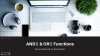### vi. MAX() and MIN() FUNCTIONS

MAX() and MIN() are two functions that likewise eliminate the need to write complex IF() statements. The functions output either the maximum or minimum values in the cells and/or ranges referenced in the formula.

So for instance, if you have a list of 10,000 values and you’d like to know what the maximum value is in the list, you’d use the MAX() function. Likewise, if you’d like to know the minimum value in that list, you’d use the MIN() function.

In real estate financial modeling, the MAX and MIN functions are key to building waterfall models.

The syntax for the MAX() function is:

MAX(number1, [number2], …)

The syntax for the MIN() function is:

MIN(number1, [number2], …)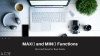### vii. COUNTIF() and COUNTA() FUNCTIONS

The next two functions involve counting ranges. COUNTIF() counts the number of values in a range that meet some specific criteria (e.g. count how many 1 bedroom units are in a list). While COUNTA() counts the number of cells in a range that are NOT empty.

These functions are especially helpful when analyzing rent rolls, performing comp analysis, or otherwise analyzing data sets in general.

The syntax for COUNTIF() is:

COUNTIF(range, criteria)

The syntax for COUNTA() is:

COUNTA(value1, [value2], …)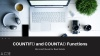### viii. IRR() and NPV() FUNCTIONS

The IRR() and NPV() functions are two finance-related functions that are common to real estate underwriting. The IRR() function calculates the discount rate at which the net present value of the investment is equal to zero. The IRR() functions assumes that the cash flows of the investment are made in regular intervals.

The NPV() function in Excel calculates both the present value of a string of irregular future cash flows as well as the net present value of an investment. To calculate the present value, simply leave out the time zero cash flow. To calculate the net present value, include the time zero cash flow.

The syntax for IRR() is as follows:

IRR(values, [guess])

The syntax for NPV() is as follows:

NPV(rate,value1,[value2],…)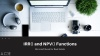### ix. EOMONTH(), EDATE(), XIRR(), AND XNPV() FUNCTIONS

The next four functions appear to be unrelated. EOMONTH and EDATE are date functions, where as XIRR and XNPV are finance functions. However, I’ve included them both in this section since they work in tandem in a real estate financial modeling context.

The EOMONTH() function returns the date for the last day of the month that is a given number of months before or after the pre-defined start date. The EDATE() function returns the date that is a given number of months before or after the pre-defined date.

XIRR() and XNPV on the other hand, are the same as the IRR() and NPV() functions only they’re able to accommodate irregular cash flow intervals. So in a real estate context, they’re used in the case of non-annual periods (e.g. monthly periods).

Since XIRR and XNPV are meant to handle irregular cash flow intervals, it’s necessary to include the dates that align with those cash flows. And thus, the use of EOMONTH and EDATE in tandem with these functions becomes necessary.

The syntax for EOMONTH() is as follows:

EOMONTH(start_date, months)

The syntax for EDATE() is as follows:

EDATE(start_date, months)

The syntax for XIRR() is as follows:

XIRR(values, dates, [guess])

The syntax for XNPV() is as follows:

XNPV(rate, values, dates)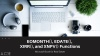### x. SUMIF() and SUMPRODUCT() FUNCTIONS

The SUMIF() and SUMPRODUCT() functions are companions to the SUM() function, providing greater flexibility to sum and multiply values. These functions are helpful in working with rent rolls, rolling up cash flows, calculating weighted averages, and much more.

SUMIF() calculates the sum of a range of values that meet a certain criteria. Alternatively, the SUMIFS() function sums a range of values that meet more than one criteria. SUMPRODUCT() returns the sum of the products of two or more corresponding ranges.

The syntax for SUMIF() is:

SUMIF(range, criteria, [sum_range])

The syntax for SUMPRODUCT() is:

SUMPRODUCT(array1, [array2], [array3], …)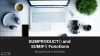### xi. PMT() and PV() FUNCTIONS

The PMT() and the PV() functions are used in modeling real estate debt. PMT() is used in real estate financial modeling to calculate the amortizing payment of a loan, while the PV() function is used to calculate the remaining loan balance at a given point in time of a loan.

The PMT() function calculates the payment for a loan based on constant payments and a constant (i.e. fixed) interest rate. The PV() function likewise assumes a constant interest rate in calculating the present value (i.e. outstanding balance) of a loan

The syntax for PMT() is:

PMT(rate, nper, pv, [fv], [type])

The syntax for PV() is:

PV(rate, nper, pmt, [fv], [type])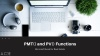### xii. IFERROR() and ISERROR() FUNCTIONS

An important discipline in real estate financial modeling is to constantly be error checking your work. There are a variety of techniques for doing this, and a handful of Excel functions to help with those techniques. IFERROR() and ISERROR() are two such functions.

IFERROR() returns a value you specify if a formula results in an error, otherwise it returns the result of that formula. ISERROR is a logic statement, that returns a TRUE if a formula (or cell) results in an error and FALSE if a formula (or cell) does not result in an error.

The syntax for IFERROR() is:

IFERROR(value, value_if_error)

The syntax for ISERROR() is:

ISERROR(value)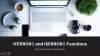### xiii. ROUND() and ROUNDUP() FUNCTIONS

Two additional functions that are important to real estate financial modeling are the ROUND() and ROUNDUP() functions. The ROUND() function rounds any value to some pre-defined number of places, where as the ROUNDUP function rounds up any value to some pre-defined number of places.

Personally, I use ROUND() to simplify the results of my analysis and for error checking purposes. I use the ROUNDUP() function to create year and quarter headers when working with monthly periods.

The syntax for ROUND() is:

ROUND(number, num_digits)

The syntax for ROUNDUP() is:

ROUNDUP(number, num_digits)### xiv. INDEX() and MATCH() FUNCTIONS

The next two functions are used together to find specific references or values within a given range of cells. So imagine you had a table of rents, by city and property type. And you wanted to dynamically output in a separate cell the rent in a user-specified city for a user-specified property type. A combination of INDEX() and MATCH() makes this possible.

Another example of using these two functions together is in modeling rent growth with rent growth assumptions that change each year. In modeling your market rent, you would use an INDEX() + MATCH() to find the user-defined rent growth value for each year and apply that to your market rent.

I should also note that this combination of INDEX() and MATCH() is a far more efficient alternative to using the dreaded VLOOKUP() and HLOOKUP() functions. Those functions slow down your Workbook and are difficult to follow/audit.

The syntax for INDEX() is:

INDEX(array, row_num, [column_num])

The syntax for MATCH() is:

MATCH(lookup_value, lookup_array, [match_type])

The syntax for INDEX() + MATCH() with one user-defined variable (e.g. rent growth) is:

INDEX(array, 1, MATCH(lookup_value, lookup_array, [match_type])) or INDEX(array, MATCH(lookup_value, lookup_array, [match_type]),1)

The syntax for INDEX() + MATCH() with two user-defined variables (e.g. rent by city and property type) is:

INDEX(array, MATCH(lookup_value, lookup_array, [match_type]), MATCH(lookup_value, lookup_array, [match_type]))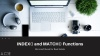### xv. CONCATENATE LOGIC

The last bit of formula logic I’ll discuss is how to concatenate values. Concatenation is a computer programing concept, that means to join character strings together. In real estate financial modeling, concatenation is an eloquent way to combined disparate inputs and values.

So for instance, imagine you had separate inputs for address, city, state, and zip code. But you wanted to display the entire address in a format that others could read and make sense of (i.e. the Address, City, State, Zip Code format). Concatenation allows you to combine those separate inputs into one.

I should mention that there are multiple ways to concatenate in Excel. The first is to use the CONCATENATE() function. The second is to simply enter a ‘&’ sign between cell references or text values to concatenate those values. My preference is to use the latter technique, as it results in shorter and simpler formulas.

In terms of syntax, imagine you had separate references for address, city, state, and zip code, and you wanted to combine those using concatenation in Excel. The syntax would read as follows:

=[Address Cell Reference]&”, “&[City Cell Reference]&”, “&[State Cell Reference]&”, “&[Zip Code Cell Reference]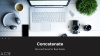## Part II – Excel Features to Master for Real Estate Financial Modeling

Microsoft Excel has been around for over 35 years. In that time, hundreds of features have been added to this ubiquitous spreadsheet tool. For a budding real estate professional just learning Excel, it can be overwhelming trying to master them all.

However, as is the case with Excel functions, in my experience there are only a handful of Excel features that truly matter to real estate financial modeling. While it certainly doesn’t hurt to learn the other features Excel offers, I regularly use the following Excel features in my own models.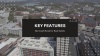### 1. DATA VALIDATION

A well-built real estate financial modeling is built on the framework of Inputs, Calculation Modules, and Outputs. Inputs (e.g. rent growth) are first entered by the user. Those inputs then run through calculation modules (e.g. operating cash flow module). The result of those calculations are shown as outputs (e.g. expense ratio by year).

It’s important as the creator of the model to guide users to enter proper values for inputs. You don’t want a user entering a non-numeric value for say, rent growth. Because that non-numeric value would then lead to an error as the value enters the calculation module.

To limit what exact inputs or what kind of inputs a user can enter, Excel has the Data Validation feature.

Data Validation restricts the type of data or the values that users enter into a cell. So for instance, with Data Validation you can limit inputs in a given cell to a specific type of value (e.g. whole numbers only). You can also restrict inputs to only pre-determined values (e.g. a drop-down menu with a specific list).

The Data Validation feature can be found by going to:

‘Data>Data Validation>Data Validation…’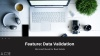### 2. IN-CELL LABELS

In the interest of building models with the end user in mind, we come to the next Excel feature I use quite often in real estate financial modeling: In-Cell Labels. Now I should mention that In-Cell Labels is part of Excel’s custom cell formatting feature. I review to this here as In-Cell Labels to differentiate this concept from the numerous other ways you can use custom cell formatting in Excel.

To create In-Cell Labels, first open the ‘Format Cells’ dialog box. Under category, select ‘Custom’. And then under type append any Type with a label wrapped in quotation marks.

So for instance, if you want the user to enter a numeric value for net rentable area (e.g. 10,000) but then you want to append their entry with the letters ‘NRA:

You would do the following:

Format Cells>Number>Category>Custom: #,##0 “NRA”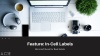### 3. CONDITIONAL FORMATTING

Another feature to help with the user experience is Excel’s Conditional Formatting feature. This feature allows you to choose custom formats for a given cell, based on some logic. So for instance, you could have a cell’s font turn blue (i.e. denote an input cell) when a user chooses a certain option in a drop-down menu. Or you could have certain cells gray out, when certain conditions don’t apply.

In short, Conditional Formatting allows you to create a custom look for certain cells (or the entire model) based on user inputs.

To create a custom Conditional Formatting rule, go to:

Home>Conditional Formatting>New Rule…>Use a formula to determine which cells to format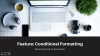### 4. DATA TABLES

The next feature is especially useful for scenario analysis in real estate, as well as displaying outputs in a dynamic way. The Data table feature is a feature in Excel that calculates multiple results (i.e. outputs) instantly. I should also point out that Data Tables really slow down your Workbook, and so it’s important to use them sparingly and/or turn the Excel calculation method to ‘Automatic Except Data Tables’.

To access the Data Table feature go to:

Data>Forecast>What-If-Analysis>Data Table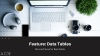### 5. GOAL SEEK

Goal seek is another useful feature to speed up analysis. Oftentimes, you’re presented with a target to hit, and you need to figure out what input value is required to hit that target. So for example, you might need to earn an 8% internal rate of return on investment and you need to quickly calculate what land value you’d have to pay to hit that 8% target. Goal seek makes this quick and simple.

To access the Goal Seek feature go to:

Data>Forecast>What-If-Analysis>Goal Seek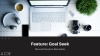### 6. CHARTING TOOLS

The final feature to bring up in this guide is Excel’s Charting Tools feature. Charting tools allows you to visually present your outputs in a compelling (and dynamic) way. Perhaps you’d like to visually display the annual net operating income of an investment. You can use a column chart to do so. Or imagine you’d like to dynamically show the returns between a variety of scenarios created using the Data Table feature. Again, charting tools makes that possible.

To simplest way to access Excel’s Charting Tools feature is to select the data you want to visualize and then go to:

Insert>Recommended Charts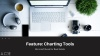## So What Comes Next?

Now that you have a basic proficiency using various Excel functions and features necessary for real estate, it’s now time to turn your attention to mastering real estate financial modeling.

Looking to fast-track your learning? Consider joining our real estate financial modeling Accelerator program

A few years back, I wrote a blog post entitled Learning Real Estate Financial Modeling in Excel. In that post, I share the pillars to real estate financial modeling mastery, namely: a sound understanding of finance, a mastery of real estate principles, and an advanced proficiency in Microsoft Excel.

You’re well on your well to mastering Excel. Now check out that post (link at beginning of proceeding paragraph) to find resources for tackling the other two pillars of real estate financial modeling mastery.

## Version Notes

v1.2

• Misc. labeling cleanups

v1.1

• Fixed issue where duplicate video had been used

v1.0

• Initial release

Tags: